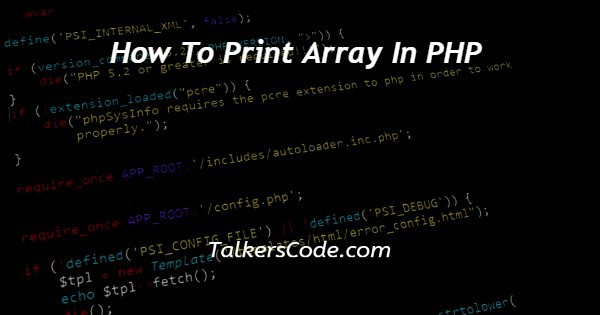# How To Print Array In PHP

Last Updated : Sep 15, 2023In this article we will show you the solution of how to print array in PHP, arrays are a flexible and popular data structure in PHP that let you store and work with many values of various data kinds.

A fundamental process called printing an array can be helpful for showing data to consumers as well as for visualising and debugging your code.

You can print an array in PHP in a variety of methods, and whatever one you use will depend on your unique needs.

In this tutorial, we’ll look at various methods for printing arrays in PHP, such as the print_r() and var_dump() functions as well as loops like foreach and for to iterate through the array.

This post will teach you how to print arrays successfully and quickly whether you’re a novice or an expert PHP developer. Now move to the concept of how to print array in php.

## Step By Step Guide On How To Print Array In PHP :-

### Method - Print an Array using for loop

```<?php
\$numbers = [1, 2, 3, 4, 5];
\$arrLength = count(\$numbers);
for (\$i = 0; \$i < \$arrLength; \$i++) {
echo \$numbers[\$i];
if (\$i < \$arrLength - 1) {
echo ", ";
}
}
?>
```
1. Here, you can see how the Python code is written to generate an indexed array of names and then use a for loop to print each of them on a separate line.
2. Using the values "John", "Mary", "Tom", & "Emma", we defined the indexed array of names at the beginning of the code using the array() function.
3. The array's size is then calculated using the count() method, and the output is saved in the \$numOfNames variable.
4. The array is then iterated over using a for loop, with each iteration beginning at index 0 and terminating at the last index, which is equal to \$numOfNames - 1.
5. The HTML <br> tag adds a line break to the output after the echo statement, which outputs the name at the current index during each iteration.
6. The PHP script stops after the for loop is finished running, and the output is then forwarded to the user's web browser.
7. Each name in the array is printed on a new line in the output, as indicated by the <br> tag.

### Method - Use echo Statement

```<?php
\$students = array("mike", "john", "rocky", "scarlett");
echo \$students . "<br>";
echo \$students . "<br>";
echo \$students . "<br>";
echo \$students . "<br>";
?>
```
1. You can see that we defined an indexed array of students in this Python code, and we used the echo command to print each element of the array on a separate line.
2. The array() function is used to define an array at the beginning of the code with the values "mike," "john," "rocky," and "scarlett."
3. An index in the array is given to each value, starting with 0.
4. Then, each element of the array is output one at a time using the echo instruction.
5. \$students prints the array's initial element, which has an index of 0.
6. The HTML line break element, denoted by "<br>," is joined to the string using the. operator.
7. The output is then updated with a new line.
8. Similar printing is done for the array's remaining elements, always using the corresponding index.

## Conclusion :-

Thus, we have successfully acquired the knowledge necessary to print an array in PHP.

Additionally, we learnt how to use PHP's arrays and loops. The code for printing an indexed array with a for loop demonstrates how to iterate through an array's items and print each one one at a time.

How to traverse over key-value pairs in an array and print them out is demonstrated in the code for printing an associative array using a foreach loop.

I hope this article on how to print array in PHP helps you and the steps and method mentioned above are easy to follow and implement.